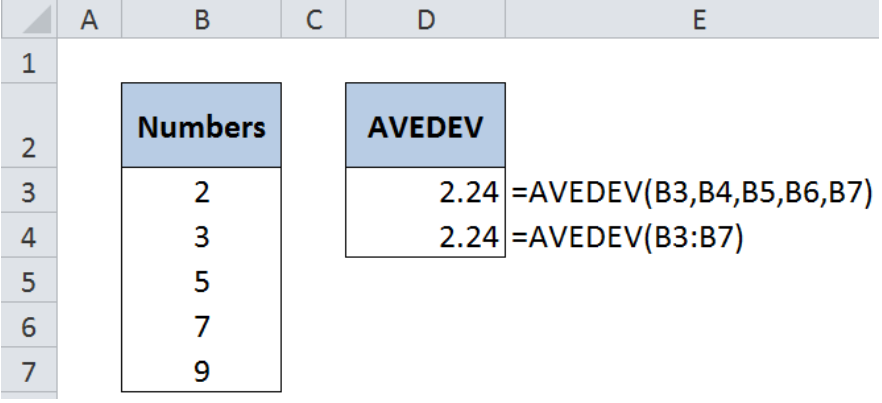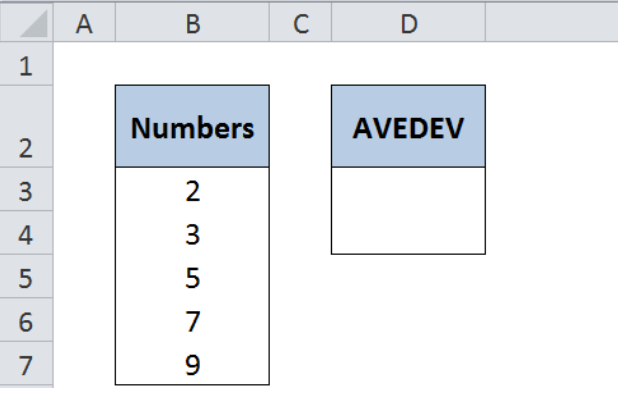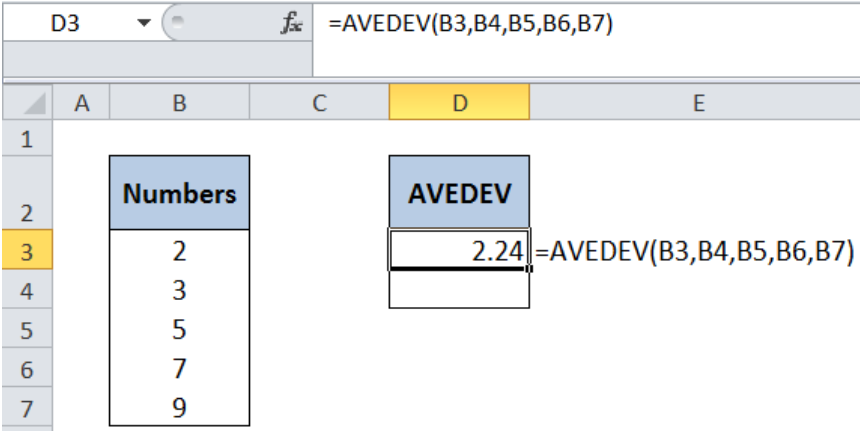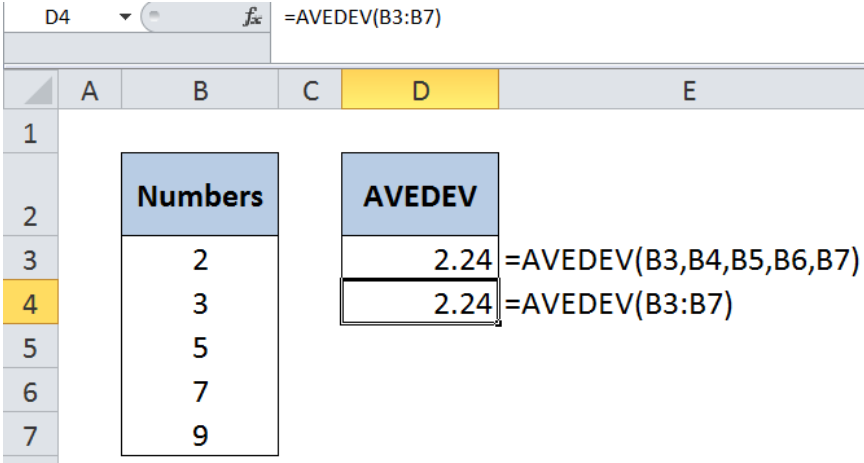Get instant live expert help with Excel or Google Sheets“My Excelchat expert helped me in less than 20 minutes, saving me what would have been 5 hours of work!”

#### Post your problem and you’ll get Expert help in seconds.

Your message must be at least 40 characters
Our professional Expert are available now. Your privacy is guaranteed.

# Excel AVEDEV Function

Excel has several built-in functions that measure variability in a data set, and AVEDEV is one of them.  AVEDEV returns the average of the absolute deviations of data points relative to the mean.  This tutorial will assist Excel users in using Avedev to measure variability of the given data.Figure 1. Final result of the AVEDEV function

## Syntax of the AVEDEV Function

`=AVEDEV(number1, [number2],...)`

Or

`=AVEDEV(array)`

The arguments of the AVEDEV function are:

• number 1, number2 – only number1 is required; subsequent numbers are optional
• The argument could also be an array; this is especially useful when dealing with large data sets, so we don’t have to input the numbers one by one

## Setting up the Data for the AVEDEV FunctionFigure 2. Sample data for use in the AVEDEV formula

The data that we will use is a list of numbers in column B, and the result for the Avedev function in column D.

## Calculate average absolute deviation using Avedev

We want to calculate the average of absolute deviations of the numbers using Avedev.

Step 1.  Select cell D3

Step 2. Enter the formula: `=``AVEDEV(B3,B4,B5,B6,B7)`

Step 3. Press Enter

The result in cell D3 is 2.24, which is the average of the absolute deviations of the numbers compared to the mean.Figure 3. Entering the formula for the AVEDEV function

## Alternative formula using an array

There is an alternative formula to calculate with Avedev using an array:

Step 1. Select cell D4

Step 2. Enter the formula: `=``AVEDEV(B3:B7)`

Step 3. Press EnterFigure 4. Using the AVEDEV formula with an array

B3:B7 is the array containing the numbers that we want to compute for average absolute deviation.  The result in cell D4 is the same as in the previous example, which is 2.24.

Most of the time, the problem you will need to solve will be more complex than a simple application of a formula or function. If you want to save hours of research and frustration, try our live Excelchat service! Our Excel Experts are available 24/7 to answer any Excel question you may have. We guarantee a connection within 30 seconds and a customized solution within 20 minutes.